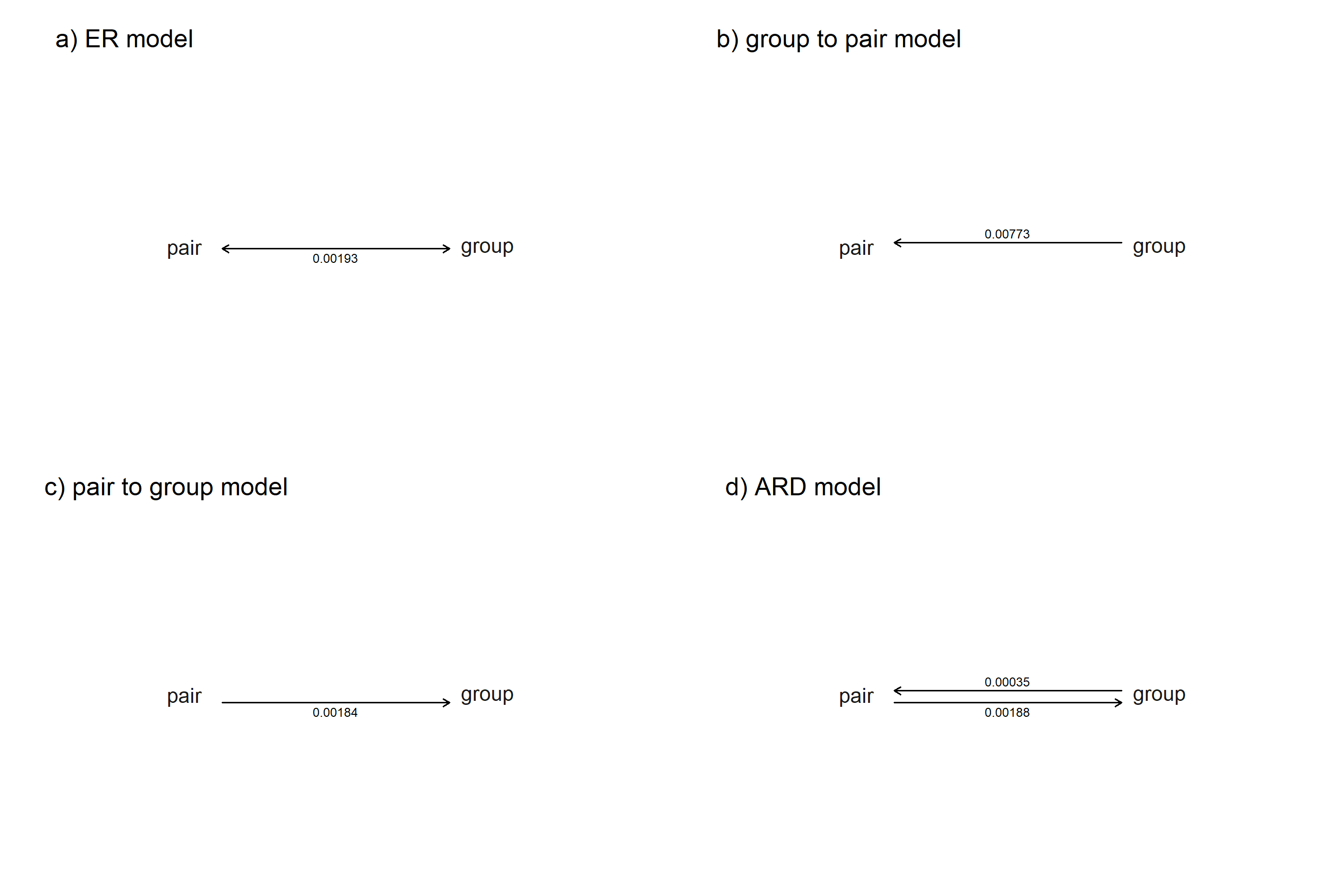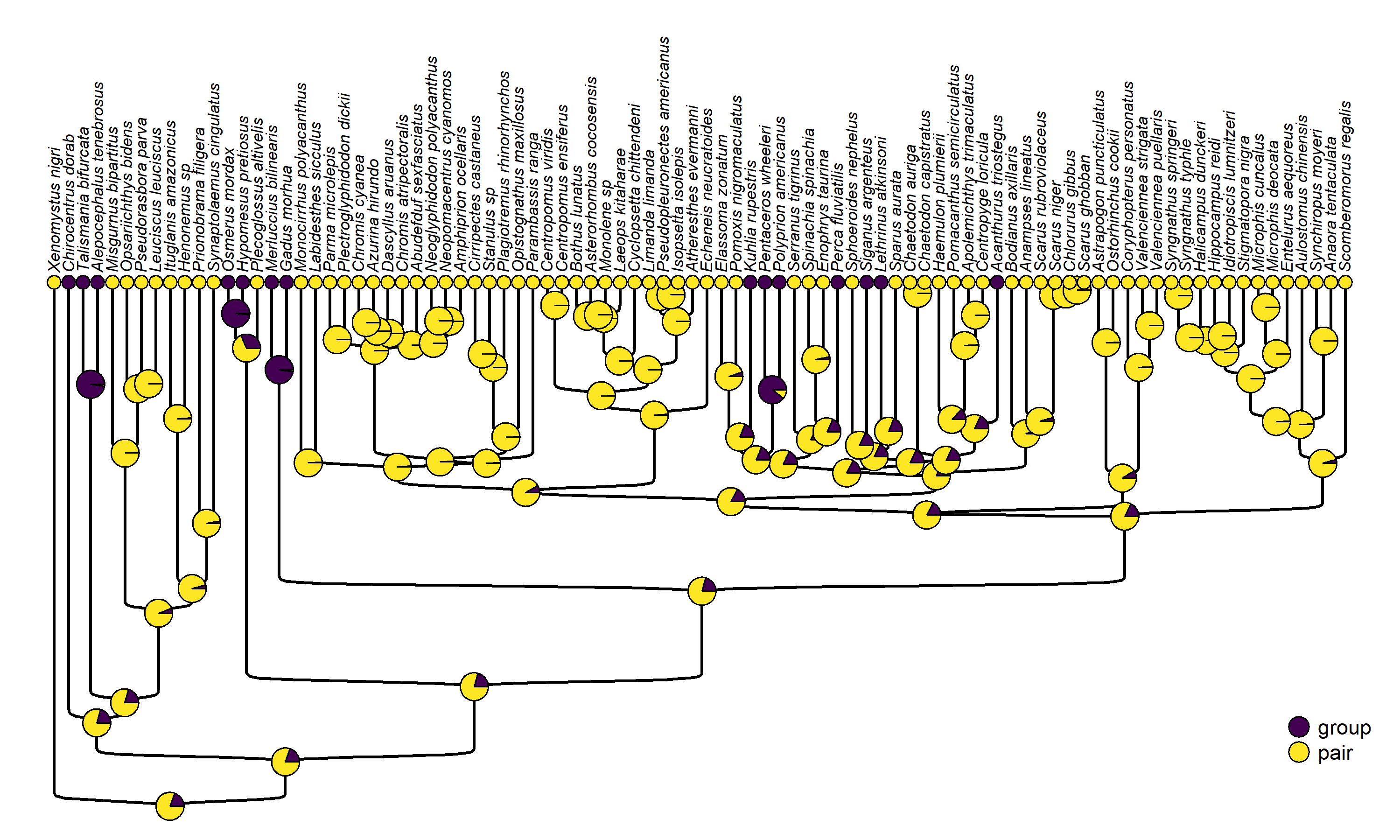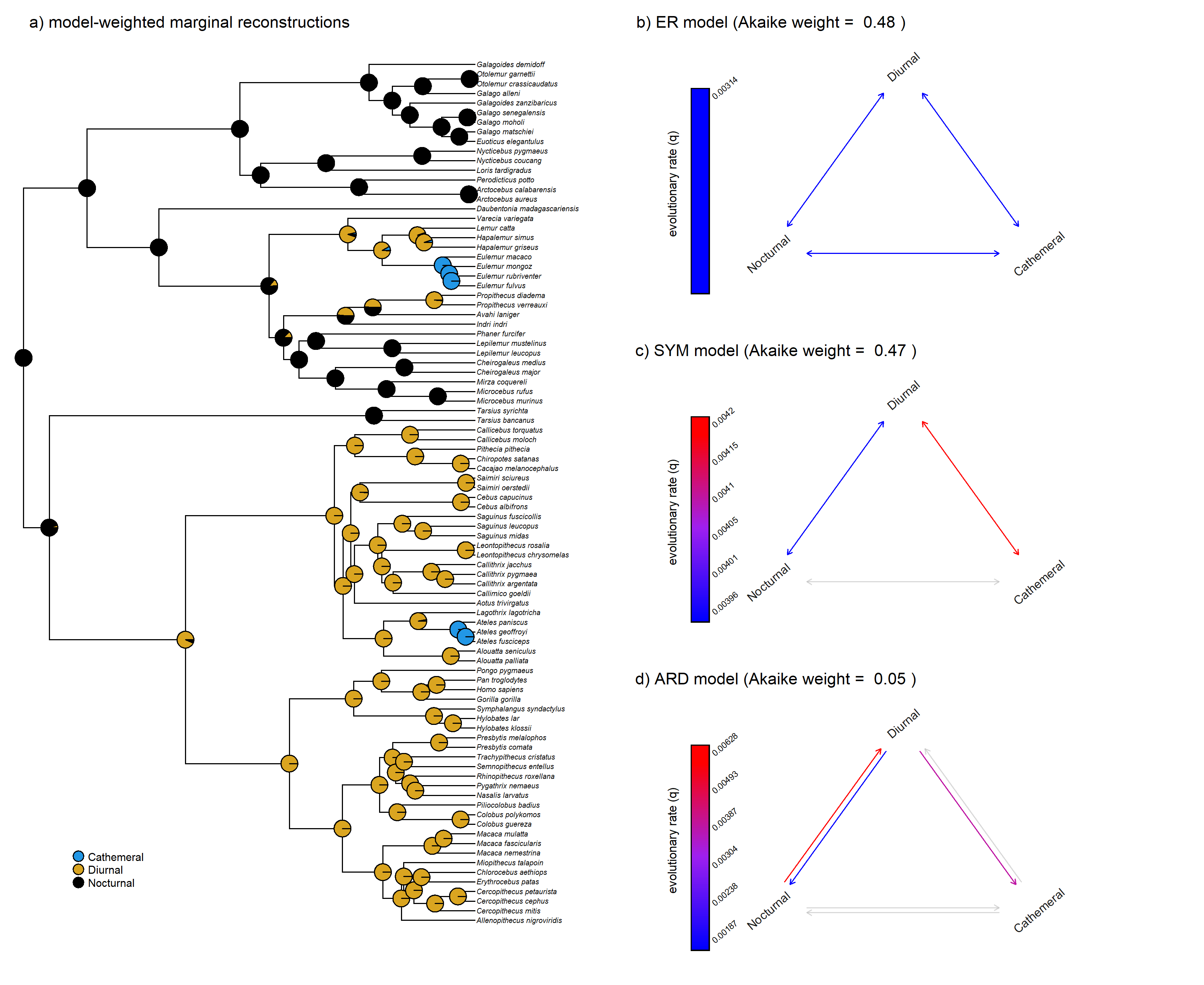## Friday, March 24, 2023

### Model-averaging in marginal ancestral state reconstruction using phytools

Over the past few days I’ve written various posts about the pruning algorithm and marginal ancestral state reconstruction for discrete characters (1, 2, 3). I implemented this in a generic method (called `ancr`) that computes marginal states based on whatever fitted model object we input!

One of the more powerful things that we might imagine doing with a method like this, however, is model-averaging – so I just added that functionality to the method. I did a similar thing for stochastic mapping last month in which different input models were sampled in proportion to their model weights. In this case, however, we can just model-weight our ancestral state estimates.

Let’s try an example based on pair vs. group spawning modes in bony fishes using a phylogeny & dataset from Benun Sutton & Wilson (2019).

``````library(phytools)
packageVersion("phytools")
``````
``````##  '1.6.2'
``````
``````data(bonyfish.tree)
data(bonyfish.data)
spawning_mode<-setNames(bonyfish.data\$spawning_mode,
rownames(bonyfish.data))
``````
``````##         Xenomystus_nigri       Chirocentrus_dorab     Talismania_bifurcata
##                     pair                    group                    group
## Alepocephalus_tenebrosus     Misgurnus_bipartitus     Opsariichthys_bidens
##                    group                     pair                     pair
## Levels: group pair
``````

For a binary character like this one, there are really only four possible extended Mk evolutionary models: equal-rates, different-rates, and the two irreversible trait evolution models (pair → group spawning, and the reverse).

Let’s fit each of these.

``````er_spawning<-fitMk(bonyfish.tree,spawning_mode,
model="ER",pi="fitzjohn")
ard_spawning<-fitMk(bonyfish.tree,spawning_mode,
model="ARD",pi="fitzjohn")
irr1_spawning<-fitMk(bonyfish.tree,spawning_mode,
model=matrix(c(0,0,1,0),2,2),pi="fitzjohn")
irr2_spawning<-fitMk(bonyfish.tree,spawning_mode,
model=matrix(c(0,1,0,0),2,2),pi="fitzjohn")
``````

We can easily plot each of our four fitted models – which is a good step to include, as a minimum to ensure that we fit our four models correctly!

``````par(mfrow=c(2,2))
mar<-c(0.1,0.1,2.1,0.1)
plot(er_spawning,spacer=0.25,
mar=mar,show.zeros=FALSE,signif=5,
offset=0.04)
plot(irr1_spawning,spacer=0.25,
mar=mar,show.zeros=FALSE,signif=5,
offset=0.04)
plot(irr2_spawning,spacer=0.25,
mar=mar,show.zeros=FALSE,signif=5,
offset=0.04)
plot(ard_spawning,spacer=0.25,
mar=mar,show.zeros=FALSE,signif=5,
offset=0.04)
``````Great! Now let’s compare our fitted models using a generic `anova` method – but (when we do) save it to a new object, `aov.spawning`.

``````aov.spawning<-anova(er_spawning,irr1_spawning,
irr2_spawning,ard_spawning)
``````
``````##                  log(L) d.f.      AIC    weight
## er_spawning   -29.51819    1 61.03638 0.2955508
## irr1_spawning -30.04038    1 62.08076 0.1753271
## irr2_spawning -29.25299    1 60.50598 0.3853082
## ard_spawning  -29.23851    2 62.47702 0.1438138
``````

We can see that the highest weight of evidence falls on the pair → group spawning irreversible model, but the result is quite ambiguous – all models received substantial support.

``````spawning_anc<-ancr(aov.spawning)
spawning_anc
``````
``````## Marginal ancestral state estimates:
##       group     pair
## 91 0.192907 0.807093
## 92 0.197408 0.802592
## 93 0.205489 0.794511
## 94 0.205445 0.794555
## 95 0.978379 0.021621
## 96 0.058689 0.941311
## ...
##
## Log-likelihood = -30.563539
``````

Finally, let’s graph this result on the tree.

``````sigmoidPhylogram(bonyfish.tree,offset=1,ftype="i",fsize=0.7,
direction="upwards")
cols_spawning<-setNames(viridisLite::viridis(n=2),
levels(spawning_mode))
nodelabels(pie=spawning_anc\$ace,piecol=cols_spawning,cex=0.5)
tiplabels(pie=to.matrix(spawning_mode[bonyfish.tree\$tip.label],
levels(spawning_mode)),piecol=cols_spawning,cex=0.25)
legend(x=0.95*Ntip(bonyfish.tree),
y=0.2*max(nodeHeights(bonyfish.tree)),
names(cols_spawning),pch=21,pt.bg=cols_spawning,pt.cex=2,
cex=0.9,bty="n")
``````Our figure shows the model-averaged marginal states – integrating across each of our four Mk models in proportion to the weight of evidence in support of each model. Cool!

Now let’s repeat this whole workflow again – but for a three-state character.

In this case, the number of possible models expands considerably – however, I’ll fit only the ER, SYM (symmetric), and ARD models. (This shouldn’t be interpreted as an endorsement of these specific models – I’ve just done this for the purposes of convenience!)

The data I’m using in this example are diel activity pattern in primates from Kirk & Kay (2004).

``````## load data
data(primate.tree)
data(primate.data)
diel_pat<-setNames(as.factor(primate.data\$Activity_pattern),
rownames(primate.data))
## fit models
er_diel<-fitMk(primate.tree,diel_pat,model="ER",
pi="fitzjohn")
sym_diel<-fitMk(primate.tree,diel_pat,model="SYM",
pi="fitzjohn")
ard_diel<-fitMk(primate.tree,diel_pat,model="ARD",
pi="fitzjohn")
## compare models
aov.diel<-anova(er_diel,sym_diel,ard_diel)
``````
``````##             log(L) d.f.      AIC     weight
## er_diel  -33.42659    1 68.85318 0.47738258
## sym_diel -31.43569    3 68.87137 0.47305890
## ard_diel -30.69175    6 73.38350 0.04955853
``````

Having fit and compared our (in this case) three models, we can go ahead and compute model-weighted marginal ancestral states using `ancr` as follows.

``````diel_anc<-ancr(aov.diel)
diel_anc
``````
``````## Marginal ancestral state estimates:
##    Cathemeral  Diurnal Nocturnal
## 91   0.000036 0.018498  0.981466
## 92   0.001103 0.047293  0.951604
## 93   0.002066 0.932075  0.065859
## 94   0.000139 0.998362  0.001499
## 95   0.000020 0.999935  0.000045
## 96   0.000008 0.999983  0.000008
## ...
##
## Log-likelihood = -33.142554
``````

Lastly, let’s plot our results.

``````## plot results
layout(matrix(c(1,1,1,2,3,4),3,2),
widths=c(0.5,0.5))
plotTree(primate.tree,fsize=0.6,ftype="i",
lwd=1,mar=c(2.1,0.1,2.1,0.1))
cols_diel<-setNames(c("#2297E6","#DAA520","black"),
levels(diel_pat))
legend(x=0.1*max(nodeHeights(primate.tree)),
y=0.1*Ntip(primate.tree),names(cols_diel),pch=21,
pt.bg=cols_diel,bty="n",cex=0.9,pt.cex=2)
nodelabels(pie=diel_anc\$ace,cex=0.7,piecol=cols_diel)
mtext("a) model-weighted marginal reconstructions",
par(srt=40)
plot(er_diel,spacer=0.25,color=TRUE,
mar=c(0.1,0.1,2.1,0.1),text=FALSE,asp=0.8,
xlim=c(-1,1),ylim=c(-1,1.2))
mtext(paste("b) ER model (Akaike weight = ",
plot(sym_diel,spacer=0.25,color=TRUE,
mar=c(0.1,0.1,2.1,0.1),text=FALSE,asp=0.8,
xlim=c(-1,1),ylim=c(-1,1.2))
mtext(paste("c) SYM model (Akaike weight = ",
plot(ard_diel,spacer=0.25,color=TRUE,
mar=c(0.1,0.1,2.1,0.1),text=FALSE,asp=0.8,
xlim=c(-1,1),ylim=c(-1,1.2))
mtext(paste("d) ARD model (Akaike weight = ",
````````````par(srt=0)
``````

That’s all there is to it.